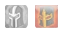Template documentation (for the above template, sometimes hidden or invisible)Copy this:
```{{Unit
|image = <gallery>

</gallery>
|unlock =
|playstyle =
|counterparts =
|resist =
|summoner =

|tname1 =
|tcost1 =
|range1 =
|as1 =
|speed1 =
|def1 =
|crit1 =
|hp1 =
|special1 =
|atk1 =
|autobuff1 =
|autodot1 =
|autodps1 =
|duration1 =
|buffchance1 =

|tname2 =
|tcost2 =
|range2 =
|as2 =
|speed2 =
|def2 =
|crit2 =
|hp2 =
|special2 =
|atk2 =
|autobuff2 =
|autodot2 =
|autodps2 =
|duration2 =
|buffchance2 =

|tname3 =
|tcost3 =
|range3 =
|as3 =
|speed3 =
|def3 =
|crit3 =
|hp3 =
|special3 =
|atk3 =
|autobuff3 =
|autodot3 =
|autodps3 =
|duration3 =
|buffchance3 =

|tname4 =
|tcost4 =
|range4 =
|as4 =
|speed4 =
|def4 =
|crit4 =
|hp4 =
|special4 =
|atk4 =
|autobuff4 =
|autodot4 =
|autodps4 =
|duration4 =
|buffchance4 =

|name1 =
|tiertype1 =
|appear1 =

|name2 =
|tiertype2 =
|appear2 =

|name3 =
|tiertype3 =
|appear3 =

|cost =
|cd =
}}```

The parameters are:

• image: an image, just the name (e.g. Skully.png), if used with gallery, put a |, followed by the name, to indicate what tier/type of unit is it
• unlock: the level that has to be beaten to unlock this unit. Levels must be manually linked.
• playstyle: the type of playstyle that this unit encourages.
• counterparts: heroes which fulfill a similar role to this unit. Heroes must be manually linked.
• resist: whether a unit resists charms or status effects in general.
• summoner: for summoned units, what unit summons them with their special.
• tname#: the name assigned to that tier.
• tcost#: the cost in moonstones to summon this unit.
• range#: the range of this unit's basic attacks.
• as#: the time, given in seconds, on how long it takes for a unit to finish their attack animation once
• speed#: speed of a unit.
• def#: defense of a unit.
• crit5#: critical chance of a unit.
• hp#: hp of a unit.
• special#: special stat of a unit.
• atk#: attack of a unit.
• autobuff#: the first status effect applied by a unit's basic attacks.
• autodot#: the second status effect, a damage over time status, applied by a unit's basic attacks.
• autodps#: if the status effect is damage over time, the amount of damage dealt per tick, which is done every second the status is active.
• duration#: the duration of the status effect.
• buffchance#: the chance to apply the status.
• name#: name of the character.
• tiertype#: tier of the character.
• appear#: first time the character appears in the story. Link to a level if applicable, if only in a book chapter, state what chapter the character appears in.
• cost: cost to train a unit during a level in gems.
• cd: cooldown to train a unit.

Visit Template:Unit/doc to edit this text! (How does this work?)